CipherJan 22, 2017

Ceres and Pluto photoshop images 1/19/2017

This will be a short post, I will be looking for Saturn tributes since both posts were released on a very relevant date

Don't Judge an Asteroid by its Cover - https://www.nasa.gov/feature/don-t-judge-an-asteroid-by-its-cover-mid-infrared-data-from-sofia-shows-ceres-true

Date numerology
1/19/2017 - 1+1+9+2+0+1+7 = 21 (Saturn = 21) - Ceres was discovered on 1/1/1801 - 1+1+18+01 = 21
1/19/2017 - 1+19+2+0+1+7 = 30 (Saturn = 10+1+2+3+9+5 = 30 - S exception)
1/19/2017 - 1+19+20+17 = 57
1/19/17 - 1+19+17 = 37
Saturn = 45+1+20+21+18+14 = 119 (Francis Bacon)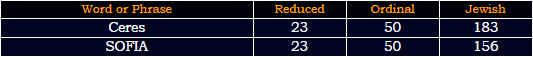156th prime number is 911, very appropriate to release news on 1/19I found something very interesting, 666th triangular number is 222,111
1503 looks a lot like 153 - 17th triangular number (God = 17), in the bible there's a miraculous catch of 153 fish

I should mention that the pictures were taken from "Stratospheric Observatory"
Stratospheric Observatory = 1+2+9+1+2+6+1+7+8+5+9+9+3+6+2+1+5+9+4+1+2+6+9+7 = 115 (Reduced)
Ceres is  in "Asteroid Belt" that is supposedly located between Mars and Jupiter
Asteroid belt = 1+10+2+5+9+6+9+4+2+5+3+2 = 58 (S Exception)
Fifty-eight = 6+9+6+20+25+5+9+7+8+20 = 115 (Ordinal)

This was said by Pierre Vernazza - research scientist from Laboratoire d'Astrophysique de Marseille (LAM-CNRS/AMU)
LAM-CNRS/AMU = 12+1+13+3+14+18+19+1+13+21 = 115 (Ordinal)
Saturn = 511 (Jewish) - 511 converted to Octal is 777, 77 converted to Octal is 115 (511+151+115 = 777)
Cube of Saturn = 3+200+2+5+50+6+90+1+100+200+80+40 = 777 (Jewish)

Pierre = 7+9+5+9+9+5 = 44 (Reduced)
Pierre = 16+9+5+18+18+5 = 71 (Ordinal)
The only 2 numbers that sum to 144 when written out (Mark of the Beast = 144, King Solomon = 144, Light = 144 (J), Time = 144(J), Two-One-Six = 144)Pierre = 60+9+5+80+80+5 = 239 (Jewish)
Torah = 239, Golden Gate = 239, Jews believe that Messiah will come through the golden gate

Vernazza = 4+5+9+5+1+8+8+1 = 41 (Reduced)

13th triangular number is 41 (USA = 41, King = 41, Globe = 41)

This picture is from the post, they are talking about composition of the Ceres... For now let's ignore the fact that you can't see the inside of something based on the surfaceEverything NASA does it by the numbers, it's the organization dedicated to worshiping Saturn mostly
anhydrous pyroxene dust = 1+14+8+25+4+18+15+21+19+16+25+18+15+24+5+14+5+4+21+19+20 = 311 (Ordinal)
Vernazza = 22+5+18+14+1+26+26+1 = 113 (Ordinal)
The date of release had 30 date numerology - 30th prime number is 113 (Universe = 113, Explorer = 113, Mainstream = 113, Green Screen = 113, Dishonest = 113)
water ice = 900+1+100+5+80+9+3+5 = 1103 (Jewish)
anhydrous pyroxene dust = 1+40+8+400+4+80+50+200+90+60+400+80+50+300+5+40+5+4+200+90+100 = 2207 (Jewish)
22/7 = 3.14Ceres was discovered by Giuseppe Piazzi on First of January in 1801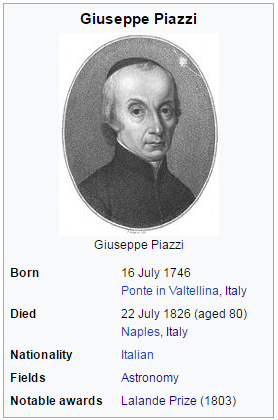From the time he found Ceres to his death is 9333 days...Very interesting that he died on 7/22 or 22/7/1826 as it is written in Europe
July Twenty Second = 1+3+3+7+2+5+5+5+2+7+10+5+3+6+5+4 = 73 (S Exception)
22/7/1826 - 22+7+18+26 = 73 (21st prime number (Saturn = 21), this post comes on 1/19/2017 with 21 date numerology)

He died in 'Naples'
Naples = 40+1+16+12+5+19 = 93 (Francis Bacon)
Saturn = 19+1+20+21+18+14 = 93 (Ordinal)

Naples = 40+1+60+20+5+90 = 216 (Jewish)
6x6x6 = 216

Now there's something interesting I found, in 2016 would be 190th anniversary of Giuseppe Piazzi's death
one hundred ninety = 6+5+5+8+3+5+4+9+5+4+5+9+5+5+2+7 = 87 (Reduced)
Piazzi = 16+9+1+26+26+9 = 87 (Ordinal)
eight seven = 5+9+7+8+100+90+5+700+5+40 = 969 (Jewish)
Speed of Saturn is 969 km/s

So if we measure from anniversary of his death in 2016 we will get 181 days (42nd prime number)
42nd triangular number is 903
Piazzi = 7+9+1+8+8+9 = 42 (Reduced)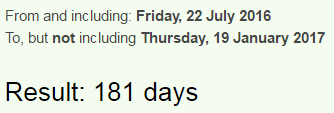one hundred ninety years = 6+5+5+8+3+5+4+9+5+4+5+9+5+5+2+7+7+5+1+9+10 = 119 (S Exception)

I just realized something while I was writing out this post

There's 3 combinations to number 119.... 911+191+119 = 1221
12/21 = 33 , but other than that 12/21 is when the time of Capricorn starts and it is ruled by Saturn til 1/19, but there's something even more interesting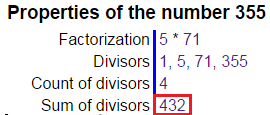December twenty first = 4+5+3+5+13+2+5+18+20+23+5+14+20+25+6+9+18+19+20 = 234 (Ordinal)
432+234 = 666

On 1/19/2017 NASA released this -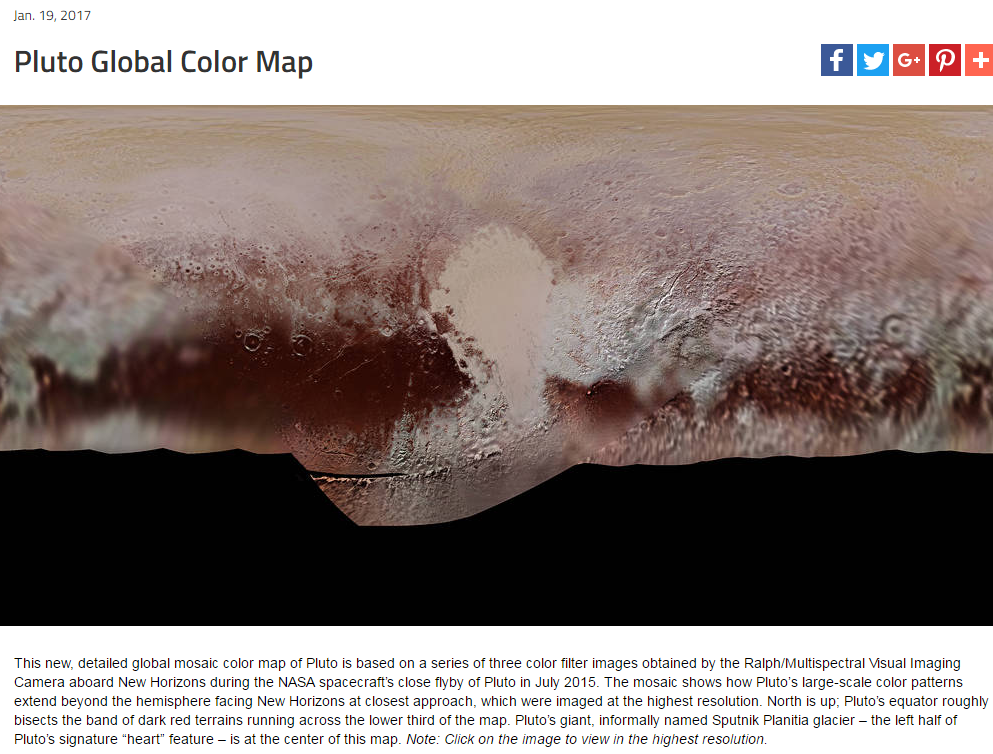Let's ignore the fact that this image is 100% made in photoshop

The title has very expected gematria connecting to 93 which is connected to 119 (1/19)
Pluto Global Color Map = 16+12+21+20+15+7+12+15+2+1+12+3+15+12+15+18+13+1+16 = 226 (Ordinal)
Two hundred twenty six = 2+5+6+8+3+5+4+9+5+4+2+5+5+5+2+7+1+9+6 = 93 (Reduced)

Saturn = 19+1+20+21+18+14 = 93 (Ordinal)
Saturn = 45+1+20+21+18+14 = 119 (Francis Bacon)
Star of David = 19+20+1+18+15+6+4+1+22+9+4 = 119 (Ordinal)
God Saturn = 7+15+4+19+1+20+21+18+14 = 119 (Ordinal)
Milky Way = 13+9+12+11+25+23+1+25 = 119 (Ordinal)
All Seeing Eye = 1+12+12+19+5+5+9+14+7+5+25+5 = 119 (Ordinal)